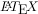### traffic lights, part two

30 April 2013

Half a decade ago, I wrote a blog post describing the optimal deceleration pattern, to maximize your velocity through a traffic light just as it turns green. It is practically illegible. Since I've been applying the calculus of variations to some ongoing research projects, redoing the math seems like some good practice.

### setup

You are driving a car towards a red light, and what do you see? An empty lane! You merge over, eagerly anticipating zooming off and laughing maniacally at the chumps who haven't even lifted their chumpy feet off of the gas pedal, you having perfectly timed the light. In a world where you know when the light will change, and your only available option is to brake before the light -— no fair braking to a stop, then goosing the throttle as the light is about to turn -— how should you manage your brake pedal so as to maximize the speed at which you coast through the intersection?

We have the following primitives:

• There is timeuntil the light changes.
• You are currently traveling at speed.
• Your available braking poweris constrained by.
• There is distancebetween your vehicle and the stop line.

### the objective

From this setup, the mathematical objective is expressed asThe calculus of variations (for an excellent reference, see Intriligator, 1971) gives us a [pointwise] Lagrangian ofSince the Lagrangian is-free, the solution may be expressed asIn particular, we haveIt is worth noting thatis a constant, as it is the multiplier on an integral constraint, whileis a function.

### analysis

As is usual in Lagrangian analysis, we look at cases.Then the integral constraint is irrelevant. It is easy to see that the solution is.We can see that. Hence if, it must be that.

In either case, the optimal deceleration pattern is given byThe question then reduces to solving for; however, at this point we have already solved as much as the previous post: the optimal strategy is to brake as hard as possible, for as long as necessary to just meet the light at the no-more-braking speed. From the above analysis, we know thatwhen.

In all other cases, we know thatsolvesIt follows that when, we have### alternative characterization

Rather than using the '-free' shortcut, we can appeal directly to the Euler equation,In this case,It is worth noting that, at almost every point,implies, and vice-versa. Hence if, it must be thatand(otherwise, we can integrate bothandslightly to positive values, implying that both constraints bind). This implies that one constraint or the other will bind throughout.

In the more interesting case,. This implies that eitheror; since the equation is set equal to, these are mutually exclusive. Remembering that each ofmust be positive, the only solution here consistent with continuousis to haveon a rangeandon a range. By the nature of the constraints binding over different regions, this implies the same solution as above.

All includedgraphics are generated at LaTeX to png .